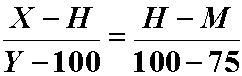HOW I CALCULATE MY GRADING CURVES

Dr. Don Stierman - updated 02/06/01

Tests can vary in difficulty. I always write fresh tests (there are many copies of old tests floating around) so the wording are order of things might vary from year to year (what might be important is unlikely to change very much but the way I ask the questions might change).

After counting up the total correct points on all tests, I set the mean point total equal to 75% and the top score equal to 100%. Exceptions: (A) if the raw mean score is greater than 75%, students keep their raw scores. I do not curve down a test percentage <note that 'points' are not the same as percentage!> . (B) If there is an outlyer at the top - one person who scores significantly above the normal group of "A" students - I use the second-best score to define "100%". (C) If the class appears, on the average, above average, the mean may be set at a higher percentage, perhaps at 80%. An "above average" class is one in which more than half of the students write "B" or better answers to short essay questions.

The rest is simply high school algebra. Y = F(X) = mx + b where X (or x) is raw points and Y is the percentage equivalent. I use the high score H and the mean score M as 2 points along that straight line. These 2 points have (x,y) coordinates of (H,100) and (M,75). The equation of the straight line that goes through these points is found by solvingfor Y.

Bonus points (if offered and awarded) are added to a student's score after the curve coefficients are calculated.

Examples of bonus questions offered in recent years:

Name one of the starting 5 Lady Rockets (basketball).

Who was Toledo's opponent in this season's Homecoming? What was the final score?

Describe one of Dr. Stierman's Web pages (other than those pages directly related to this course).

Bonus questions often deal with university activities - things students who are participating in a full college experience should be familiar with. Because bonus points are not used when calculating the curve, a person who can not answer a bonus is not penalized. However, a person who does answer correctly wins a few points. In one recent test, the top score after bonus was 105%.